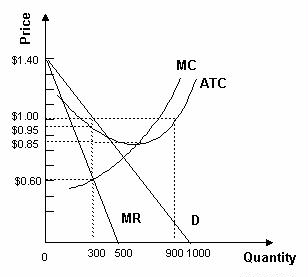### CFA Practice Question

There are 923 practice questions for this topic.

### CFA Practice Question

Refer to the graph below. The monopolistically competitive firm is currently producing 300 units of output. The firm is in ______A. both short-run and long-run equilibrium because price exceeds average total cost at the profit-maximizing output level.
B. short-run equilibrium because price exceeds average total cost at the profit-maximizing output level.
C. long-run equilibrium because economic profits are zero at the profit-maximizing output level.

Since economic profits are positive at the profit-maximizing output level and there are no barriers to entry in a monopolistically competitive industry, this industry cannot be in long-run equilibrium.

User Comment
danlan In long-run equilibrium, economic profits are zero.
ridone The price is 1.00 and the average cost is 0.95.
CFAonTheBrain can someone explain the diffrence between short and long run equilibrium again please im a little lost
YOUCANDOIT ^^^
In the short run, equilibrium is achieved as long as firm produces at an output where there MR=MC (ex.300 units). Economic profits are available as long as P>ATC.

In the long run, new firms will enter industry if profits are available. As new firms enter the industry, demand for your products will go down. (Think T-shirt companies, if Adidas is a new entrant, demand for Nike T-shirts will go down). D-Curve shifts leftward until P=ATC, where MC=MR and no economic profits are available.
YOUCANDOIT ...as a result, the price and quantity of firm's products demanded will both decrease in this example.
Teeto How do I know the price from the question?
fzhou @Teeto, according to the reading LOS, P1 in the first figure is decided by demand curve. So you take the units given in this question which is 300, find the price point lying on demand curve - this is how you can get the price.
Tashen111 @Teeto
Step 1: Look for the value of Q* where MR=MC... This is the Quantity that you will produce your goods at
Step 2: Determine the point on the demand curve where you will be producing this quantity
Step3: The price in line with that point on the demand curve is the price!!!